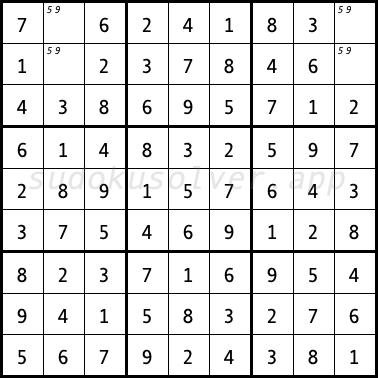# Unique Rectangle

The Unique Rectangle technique uses the fact that the sudoku has exactly one solution. If there is no guarantee that the sudoku has exactly one solution, this technique cannot be used. The following sudoku has two solutions. The four cells in the first and second rows and the second and ninth columns can be filled in two ways. These cells form the so-called non-unique rectangle, that is, four cells, which are located exactly in two rows and two columns of two boxes, and all of these cells have exactly the same two candidates. Techniques based on the unique rectangle prevents the occurrence of a non-unique rectangle.Types of techniques based on a unique rectangle:
Type 1
Type 2
Type 3
Type 4